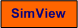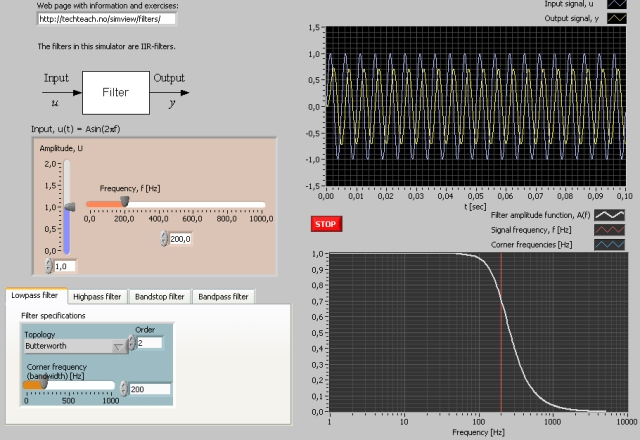# Filter Types

Snapshot of the front panel of the simulator:## Description of the system to be simulated

This simulator simulates the following types of signal filters:

• lowpass filter
• highpass filter
• bandstop filter
• bandpass filter

You can select among these filter types. You can adjust the corner frequencies and the order while the simulator runs. The input signal is a sinusoid of which you can adjuste the amplitude and the frequency, and the filter response (output) is plotted in the same diagram. In addition the amplitude gain function of the frequency response is plotted. In other words, both time domain and frequency domain properties are shown.

## Aims

The aims of the tasks below are demonstrate characteristics of various filters by showing both time domain and frequency domain properties.

## Motivation

Filters are used to attenuate (ideally: remove) certain frequency commponents of a signal. For example, lowpass filters are used to attenuate high frequent noise in a measurement signal.

The tasks below assumes that the simulator runs. Initially, you can use the default choices for filter topology, which is Butterworth, and the default choice of filter order. The input signal amplitude can be set to U = 1.

1. Lowpass filter: The filter bandwidth or corner frequency can be set to fb = 100Hz.
1. Explain from the amplitude gain function A(f) that the filter performs lowpass filtering.
2. Change the signal freqency f continuously. Does the time response confirm the lowpass characteristic of the filter (qualitatively)?
3. Set the signal freqency to a fixed value, e.g. f = 100Hz. What is the amplitude gain at this frequency as seen in the Bode plot? Is this result confirmed by the time response?
4. Select a filter order larger than the default value. How does the filtering properties depend on the filter order (observe both the amplitude function and the time response).

2. Highpass filter: Set the filter corner frequency to fc = 100Hz.
1. Explain from the amplitude function A(f) that the filter performs highpass filtering.
2. Change the signal freqency f continuously. Does the time response confirm the highpass characteristic of the filter (qualitatively)?
3. Select a filter order larger than the default value. How does the filtering properties depend on the filter order (observe both the amplitude function and the time response).

3. Bandstop filter: Set the lower corner frequency to 100Hz and the upper corner frequency to 400Hz.
1. Explain from the amplitude function A(f) that the filter performs bandstop filtering.
2. Change the signal freqency f continuously. Does the time response confirm the bandpass characteristic of the filter (qualitatively)?
3. Select a filter order larger than the default value. How does the filtering properties depend on the filter order (observe both the amplitude function and the time response).

4. Bandpass filter: Set the lower corner frequency to 100Hz and the upper frequency to 400Hz.
1. Explain from the amplitude gain function A(f) that the filter performs bandstop filtering.
2. Change the signal freqency f continuously. Does the time response confirm the bandpass characteristic of the filter (qualitatively)?
3. Select a filter order larger than the default value. How does the filtering properties depend on the filter order (observe both the amplitude function and the time response).

[SimView] [TechTeach]

Updated 27 August 2017. Developed by Finn Haugen. E-mail: finn@techteach.no.